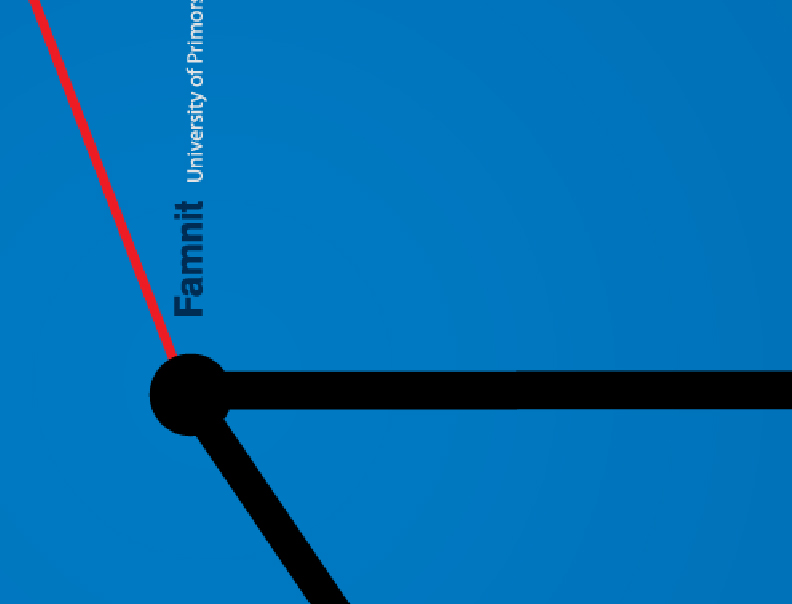Univerza na Primorskem Fakulteta za matematiko, naravoslovje in informacijske tehnologijeSI | EN# Raziskovalni matematični seminar - Arhiv

 2020 2019 2018 2017 2016 2015 2014 2013 2012 2011 2010
 1 2 3 4 5 6 7 8 9 10 11 12

28.11.2011 Lecturer: dr. Štefko Miklavič

Title: Automorphism groups of the "Rose-window" graphs which are not edge-transitive.

Title:  Quasi m-Cayley graphs

Abstract:  A graph Γ is called a quasi m-Cayley graph on a group G if there exists a vertex
∞ ∈ V (Γ) and a subgroup G of the vertex stabilizer Aut(Γ) of the vertex ∞ in the full
automorphism group Aut(Γ) of Γ, such that G acts semiregularly on V (Γ)\{∞} with m
orbits. If the vertex ∞ is adjacent to only one orbit of G on V (Γ)\{∞}, then Γ is called
a strongly quasi m-Cayley graph on G.We will present the classiﬁcations of quasi 2-
Cayley, strongly quasi 3-Cayley and strongly quasi 4-Cayley arc-transitive circulants.

14.11.2011 Lecturer: dr. Martin Milanič

Title:  Hereditary efficiently dominatable graphs

Abstract: An efficient dominating set (or perfect code) in a graph is a set of vertices the closed neighborhoods of which partition the graph's vertex set. It is NP-complete to determine whether a given graph contains an efficient dominating set. We study the class of hereditary
efficiently dominatable graphs, that is, graphs every induced subgraph of which contains an efficient dominating set. Based on a decomposition theorem for (bull, fork, C_4)-free graphs, we derive the forbidden induced subgraph characterization of hereditary efficiently dominatable graphs. We also present a polynomial time algorithm for finding an efficient dominating set (if one exists) in a class of graphs properly containing the class of hereditary efficiently dominatable graphs, by reducing the problem to the maximum weight independent set problem in claw-free graphs.